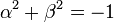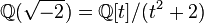# Minimal splitting field need not be unique

## Statement

### In characteristic zero

Let$G$ be a finite group. It is possible for$G$ to have two distinct non-isomorphic minimal splitting fields$K$ and$L$ in characteristic zero. In other words, both$K$ and$L$ are splitting fields, no proper subfield of either is a splitting field, and$K$ is not isomorphic to$L$.

### In prime characteristic

Not sure whether there are examples here.

## Proof

### Example of the quaternion group

The quaternion group of order eight has many different minimal splitting fields in characteristic zero. Specifically the following are true:

•$\mathbb{Q}$ is not a splitting field.
• Any field of the form$\mathbb{Q}(\alpha,\beta)$ where$\alpha^2 + \beta^2 = -1$ is a splitting field.

Thus, any field of the form$\mathbb{Q}(\sqrt{-m^2 - 1}) = \mathbb{Q}[t]/(t^2 + m^2 + 1)$, where$m \in \mathbb{Q}$, is a quadratic extension of$\mathbb{Q}$ satisfying the condition for being a splitting field, and hence is a minimal splitting field. There are multiple non-isomorphic fields of this type, such as$\mathbb{Q}(i) = \mathbb{Q}[t]/(t^2 + 1)$ and$\mathbb{Q}(\sqrt{-2}) = \mathbb{Q}[t]/(t^2 + 2)$.